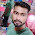### Program 315: Sum Series of 1^1/1!+2^2/2!+3^3/3!.. upto n terms

Program 315: Sum Series of 1^1/1!+2^2/2!+3^3/3!.. upto n terms

```#include<stdio.h>
#include<math.h>
int factorial(int);
main()
{
int i,n;
float sum=0;
printf("Enter value of n\n");
scanf("%d",&n);

for(i=1;i<=n;i++)
{
sum+=(float)(pow(i,i)/factorial(i));
}
printf("The value of\n");
for(i=1;i<=n;i++)
{
if(i<n)
{
printf("%d^%d/%d!+ ",i,i,i);
}
else
{
printf("%d^%d/%d!= ",i,i,i);
}

}
printf("%f\n",sum);

}

int factorial(int num)
{
int i,fact=1;
for(i=1;i<=num;i++)
{
fact=fact*i;
}

return(fact);
}
```
Explanation:

//Coming Soon...

Output:

#### 1 comment:

1.college-party-guide One of the most important rituals for students in college is to throw the most memorable student dorm celebration.
factorial hundred In the last few days, the “factorial of 100” is one of the top subjects and a lot of maths geeks compute it using voice assistants such as Alexa, Shiri, etc.
factorial hundred In the last few days, the “factorial of 100” is one of the top subjects and a lot of maths geeks compute it using voice assistants such as Alexa, Shiri, etc.
gigolo The term”gigolo” usually refers to the person who lives an arranged lifestyle that includes many of these relationships in a series, rather than having any other support system.